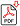Search for Articles:

## Trace of Negative Integer Power of Real 2x2 Matrices

Abstract: The purpose of this paper is to discuss the theorems for the trace of any negative integer power of 2 × 2 real Matrix. We obtain a new formula to compute trace of any negative integer power of 2 × 2 real matrix A, in the terms of Trace of A (Tr A) and Determinant of A (Det A), which are based on definition of trace of matrix and multiplication of the matrix n times, where n is Negative integer and this formula gives some corollary for Tr A^n when TrA or DetA are zero.

Keywords: Trace, Determinant, Matrix Multiplication

Country: India, Subject Area: Mathematics

Pages: 1682 - 1683

Edition: Volume 8 Issue 7, July 2019